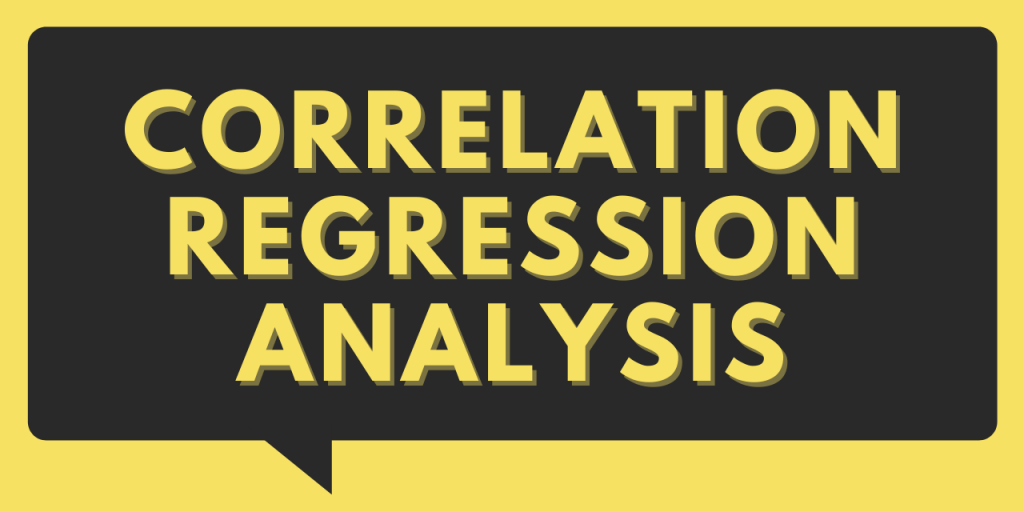# Correlation Regression Analysis in Python – 2 Easy Ways!Hello, readers! Today, we will be focusing on Correlation Regression Analysis in Python.

So, let us begin!

## First, what is Correlation amongst variables?

Let us try to understand the concept of Correlation in the context of Data Science and Machine Learning!

In the domain of Data Science and Machine Learning, the primary step is to analyze and clean the data for further processing.

In the pretext of data pre-processing, it is very important for us to know the impact of every variable/column on the other variables as well as on the response/target variable.

This is when Correlation regression Analysis comes into the scene!

Correlation Regression Analysis is a technique through which we can detect and analyze the relationship between the independent variables as well as with the target value.

By this, we try to analyze what information or value do the independent variables try to add on behalf of the target value.

Usually, correlation analysis works for regression values i.e. continuous (numeric) variables and it is depicted through a matrix known as a correlation matrix.

In the Correlation matrix, the relationship between variables is a value between range -1 to +1.

Using Correlation analysis, we can detect the redundant variables i.e. the variables that represent the same information for the target value.

If two variables are highly correlated, it gives us a heads up to eliminate either of the variables as they depict the same information.

Let us now implement the concept of Correlation Regression!

## Correlation Regression Analysis using Pandas module

In this example, we have made use of the Bank Loan dataset to determine the correlation matrix for the numeric column values. You can find the dataset here!

1. Initially, we will load the dataset into the environment using pandas.read_csv() function.
2. Further, we will segregate the numeric columns into a different Python list (variable) as shown in the below example.
3. Now, we would apply `corr() function` on every numeric variable and create a correlation matrix for the same output of this function.

Example:

```import os
import pandas as pd
import numpy as np

numeric_col = ['age', 'employ', 'address', 'income', 'debtinc', 'creddebt', 'othdebt']

#Using Correlation analysis to depict the relationship between the numeric/continuous data variables
corr = data.loc[:,numeric_col].corr()
print(corr)
```

Output:

## Using NumPy module to determine correlation between variables

The corr() method isn’t the only one that you can use for correlation regression analysis. We have another function for calculating correlations.

Python NumPy provides us with `numpy.corrcoef()` function to calculate the correlation between the numeric variables.

Syntax:

```numpy.corrcoef(col1, col2)
```

As a result, it would return a correlation matrix for the input regression variables.

Example:

```import numpy as np

x = np.array([2,4,8,6])
y = np.array([3,4,1,6])

corr_result=np.corrcoef(x, y)

print(corr_result)
```

Output:

```[[ 1.         -0.24806947]
[-0.24806947  1.        ]]
```

## Conclusion

By this, we have come to the end of this topic. For more such posts related to Python, Stay tuned!! Try implementing the concept of Correlation Analysis on different data sets and do let us know your experience in the comment section 🙂

Till then, Happy Learning!! 🙂